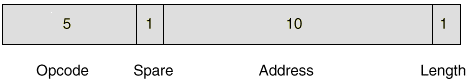## Instruction Format

Although the basic word size of the EDSAC was 18 bits, only 17 bits could actually be used due to circuit set-up times. The opcode (i.e. one of the instruction codes explained below) was specified in 5 bits, and the address in 10 bits (giving an address range of 1024 short words). The length bit specified the operand length : most instructions could operate on either a 17-bit short word (length = 0), or a 35-bit full word (length = 1).### Instruction Codes

The EDSAC Instruction Set 1949

 A n Add the number in storage location n into the accumulator S n Subtract the number in storage location n from the accumulator H n Copy the number in storage location n into the multiplier register V n Multiply the number in storage location n by the number in the multiplier register and add the product into the accumulator N n Multiply the number in storage location n by the number in the multiplier register and subtract the product from the accumulator T n Transfer the contents of the accumulator to storage location n and clear the accumulator U n Transfer the contents of the accumulator to storage location n and do not clear the accumulator C n Collate [logical and] the number in storage location n with the number in the multiplier register and add the result into the accumulator R 2^(n-2) Shift the number in the accumulator n places to the right L 2^(n-2) Shift the number in the accumulator n places to the left E n If the sign of the accumulator is positive, jump to location n; otherwise proceed serially G n If the sign of the accumulator is negative, jump to location n; otherwise proceed serially I n Read the next character from paper tape, and store it as the least significant 5 bits of location n O n Print the character represented by the most significant 5 bits of storage location n F n Read the last character output for verification X No operation Y Round the number in the accumulator to 34 bits Z Stop the machine and ring the warning bell

### Number Format

The were four number formats which could be used in the EDSAC: short and long integers, and short and long fractions.

Short numbers were 17-bits in length (for reasons explained above) and long ones 35-bits.

Whereas the multiplier and multiplicand registers each had a capacity of 35 bits, the accumulator provided 71 bits. This means that long numbers can be multiplied without overflow.### Character Codes

 Editor Window Output Window Binary Decimal Lettershift Figureshift Lettershift Figureshift P 0 P 0 00000 0 Q 1 Q 1 00001 1 W 2 W 2 00010 2 E 3 E 3 00011 3 R 4 R 4 00100 4 T 5 T 5 00101 5 Y 6 Y 6 00110 6 U 7 U 7 00111 7 I 8 I 8 01000 8 O 9 O 9 01001 9 J J 01010 10 Pi (type in as "#") Figure shift 01011 11 S S " 01100 12 Z Z + 01101 13 K K ( 01110 14 Erase (type in as "*") Letter shift 01111 15 Blank Tape (type in as "." ) (no effect) 10000 16 F F \$ 10001 17 Theta (type in as "@") Carriage return 10010 18 D D ; 10011 19 Phi (type in as "!") Space 10100 20 H + H £ 10101 21 N - N , 10110 22 M M . 10111 23 Delta (type in as "&") Line feed 11000 24 L L ) 11001 25 X X / 11010 26 G G # 11011 27 A A - 11100 28 B B ? 11101 29 C C : 11110 30 V V = 11111 31